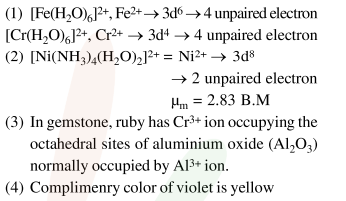# The INCORRECT statement is :

Question:

The INCORRECT statement is :

1. the spin-only magnetic moments of $\left[\mathrm{Fe}\left(\mathrm{H}_{2} \mathrm{O}\right)_{6}\right]^{2+}$ and and $\left[\mathrm{Cr}\left(\mathrm{H}_{2} \mathrm{O}\right)_{6}\right]^{2+}$ are nearly similar.

2. the spin-only magnetic moment of $\left[\mathrm{Ni}\left(\mathrm{NH}_{3}\right)_{4}\left(\mathrm{H}_{2} \mathrm{O}\right)_{2}\right]^{2+}$ is $2.83 \mathrm{BM}$.

3. the gemstone, ruby, has $\mathrm{Cr}^{3+}$ ions occupying the octahedral sites of beryl.

4. the color of $\left[\mathrm{CoCl}\left(\mathrm{NH}_{3}\right)_{5}\right]^{2+}$ is violet as it absorbs the yellow light.

Correct Option: , 3

Solution: